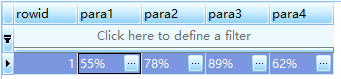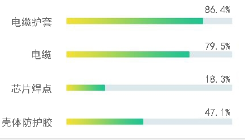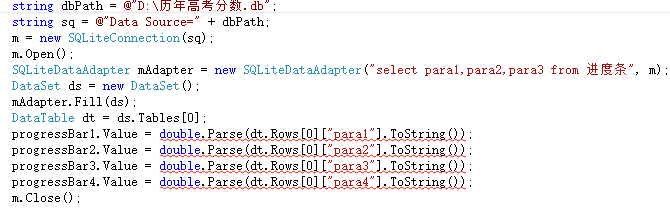C#中进度条连接数据库，并在label中显示，效果如下图，下面代码的数据源应该如何绑定，这里需要哪些引用using System;
using System.Collections.Generic;
using System.ComponentModel;
using System.Data;
using System.Data.SQLite;
using System.Drawing;
using System.Linq;
using System.Text;
using System.Windows.Forms;

string dbPath = @"D:\历年高考分数.db";
string dbPath = @"D:\历年高考分数.db";
string sq = @"Data Source=" + dbPath;
m = new SQLiteConnection(sq);
m.Open();
DataSet ds = new DataSet();
DataTable dt = ds.Tables;
progressBar1.Value = double.Parse(dt.Rows["para1"].ToString());
progressBar2.Value = double.Parse(dt.Rows["para2"].ToString());
progressBar3.Value = double.Parse(dt.Rows["para3"].ToString());
progressBar4.Value = double.Parse(dt.Rows["para4"].ToString());
m.Close();1个回答

DataTable dt = ds.Tables;
progressBar1.Value = double.Parse(dt.Rows["para1"].ToString());
progressBar2.Value = double.Parse(dt.Rows["para2"].ToString());
progressBar3.Value = double.Parse(dt.Rows["para3"].ToString());